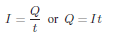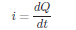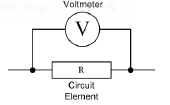ELECTRICAL ENGINEERING: The typical curriculum of an undergraduate electrical engineering student includes the subjects listed in Table 1.1. Although the distinction between some of these subjects is not always clear-cut, the table is sufficiently representative to serve our purposes. The aim of this book is to introduce the non-electrical engineering student to those aspects of electrical engineering that are likely to be most relevant to his or her professional career. Virtually all of the topics of Table 1.1 will be touched on in the book, with varying degrees of emphasis. The following example illustrates the pervasive presence of electrical, electronic, and electromechanical devices and systems in a very common application: the automobile. As you read Table 1.1 Electrical through the example, it will be instructive to refer to Table 1.1.

Table 1.1

ELECTRICAL ENGINEERING

• Circuit analysis
• Electromagnetics
• Solid-state electronics
• Electric machines
• Electric power systems
• Digital logic circuits
• Computer systems
• Communication systems
• Electro-optics
• Instrumentation systems
• Control systems

Current:

Current can be defined as the motion of charge through a conducting material. The unit of current is Ampere whilst charge is measured in Coulombs.

Definition of an Ampere:
“The quantity of total charge that passes through an arbitrary cross section of a conducting material per unit second is defined as an Ampere.”

Mathematically,where Q is the symbol of charge measured in Coulombs (C), I is the current in amperes (A) and t is the time in seconds (s). The current can also be defined as the rate of charge passing through a point in an electric circuit i.e.A constant current (also known as direct current or DC) is denoted by the symbol I whereas a timevarying current (also known as alternating current or AC) is represented by the symbol i or i(t).

Voltage or Potential Difference:
Voltage or potential difference between two points in an electric circuit is 1 V if 1 J (Joule) of energy is expended in transferring 1 C of charge between those points.

It is generally represented by the symbol V and measured in volts (V). Note that the symbol and the unit of voltage are both denoted by the same letter, however, it rarely causes any confusion.

Voltage is always measured across a circuit element as demonstrated in given figureCircuit Loads: A load generally refers to a component or a piece of equipment connected to the output of an electric circuit.

circuit elements

• 1. Resistor (R)
• 2. Inductor (L)
• 3. Capacitor (C)